# HSSlive: Plus One & Plus Two Notes & Solutions for Kerala State Board

## AP Board Class 7 Maths Chapter 14 Understanding 3D and 2D Shapes InText Questions Textbook Solutions PDF: Download Andhra Pradesh Board STD 7th Maths Chapter 14 Understanding 3D and 2D Shapes InText Questions Book AnswersAP Board Class 7 Maths Chapter 14 Understanding 3D and 2D Shapes InText Questions Textbook Solutions PDF: Download Andhra Pradesh Board STD 7th Maths Chapter 14 Understanding 3D and 2D Shapes InText Questions Book Answers

## Andhra Pradesh State Board Class 7th Maths Chapter 14 Understanding 3D and 2D Shapes InText Questions Books Solutions

 Board AP Board Materials Textbook Solutions/Guide Format DOC/PDF Class 7th Subject Maths Chapters Maths Chapter 14 Understanding 3D and 2D Shapes InText Questions Provider Hsslive

## How to download Andhra Pradesh Board Class 7th Maths Chapter 14 Understanding 3D and 2D Shapes InText Questions Textbook Solutions Answers PDF Online?

2. Click on the Andhra Pradesh Board Class 7th Maths Chapter 14 Understanding 3D and 2D Shapes InText Questions Answers.
3. Look for your Andhra Pradesh Board STD 7th Maths Chapter 14 Understanding 3D and 2D Shapes InText Questions Textbooks PDF.
4. Now download or read the Andhra Pradesh Board Class 7th Maths Chapter 14 Understanding 3D and 2D Shapes InText Questions Textbook Solutions for PDF Free.

## AP Board Class 7th Maths Chapter 14 Understanding 3D and 2D Shapes InText Questions Textbooks Solutions with Answer PDF Download

Find below the list of all AP Board Class 7th Maths Chapter 14 Understanding 3D and 2D Shapes InText Questions Textbook Solutions for PDF’s for you to download and prepare for the upcoming exams:

Question 1.
Estimate the number of cubes in the following arrangement. (Page No. 273)
Solution: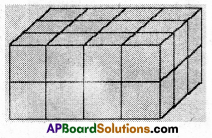4 × 3 × 2 = 24 No. of cubes in the above fig. = 24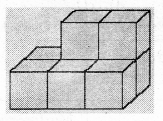3 × 2 + 2 = 6 + 2 = 8
No. of cubes in the above fig. = 8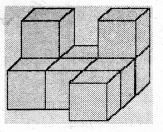3 + 2 + 2 + 1 + 1 = 9
No. of cubes in the above fig. = 9

Question 2.
Two dice are placed side by side as shown. Can you say what the total would be on the faces opposite to them. (Page No. 274)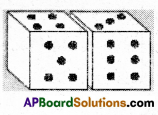i) 5 + 6 ii) 4 + 3
Solution:
i) Sum of the opposite faces = 2 + 1 = 3
ii) Sum of the opposite faces = 3 + 4 = 7

Question 3.
Three cubes each with 2 cm are placed side by side to form a cuboid. Try to make an oblique sketch and say what could be its length, breadth and height. (Page No. 274)
Solution: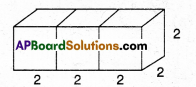Length =2 + 2 + 2 = 6cm
Height = 2 cm

Do This

Question 1.
Make clay (or plasticine) models of the following solids and make vertical or horizontal cuts. Draw rough sketches of the cross-sections you obtain. Name them if possible. (Page No. 274)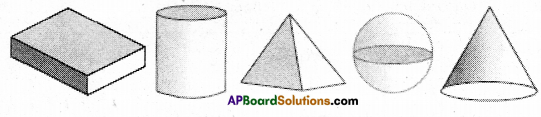Solution: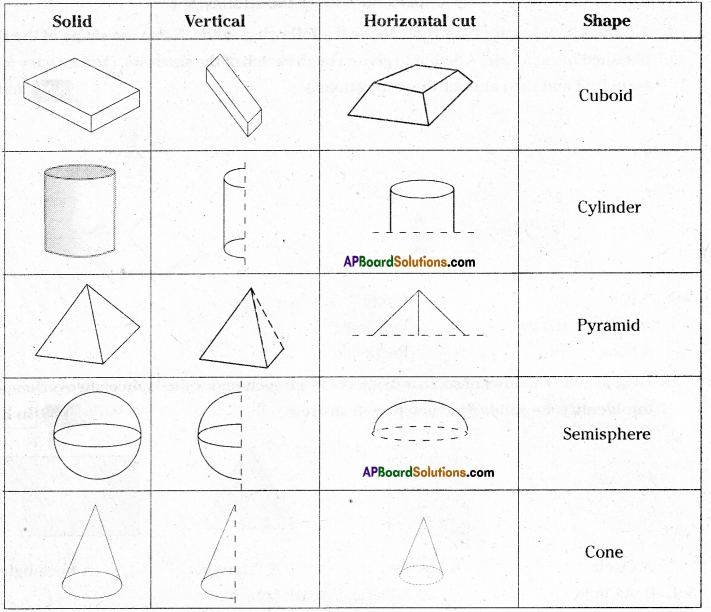Question 2.
What cross-section do you get when you give a i) vertical cut ii) horizontal cut to the following solids. (Page No. 275)
a) A brick
b) A round apple
c) A die
d) A circular pipe
e) An ice-cream cone
Solution:

 Solid Vertical cut Horizontal cut Brick Square/rectangle Rectangle A round apple Circle Circle Die Square Square Circular pipe Rectangle Circle ice-cream cone Triangle Circle

## Andhra Pradesh Board Class 7th Maths Chapter 14 Understanding 3D and 2D Shapes InText Questions Textbooks for Exam Preparations

Andhra Pradesh Board Class 7th Maths Chapter 14 Understanding 3D and 2D Shapes InText Questions Textbook Solutions can be of great help in your Andhra Pradesh Board Class 7th Maths Chapter 14 Understanding 3D and 2D Shapes InText Questions exam preparation. The AP Board STD 7th Maths Chapter 14 Understanding 3D and 2D Shapes InText Questions Textbooks study material, used with the English medium textbooks, can help you complete the entire Class 7th Maths Chapter 14 Understanding 3D and 2D Shapes InText Questions Books State Board syllabus with maximum efficiency.

## FAQs Regarding Andhra Pradesh Board Class 7th Maths Chapter 14 Understanding 3D and 2D Shapes InText Questions Textbook Solutions

#### Can we get a Andhra Pradesh State Board Book PDF for all Classes?

Yes you can get Andhra Pradesh Board Text Book PDF for all classes using the links provided in the above article.

## Important Terms

Andhra Pradesh Board Class 7th Maths Chapter 14 Understanding 3D and 2D Shapes InText Questions, AP Board Class 7th Maths Chapter 14 Understanding 3D and 2D Shapes InText Questions Textbooks, Andhra Pradesh State Board Class 7th Maths Chapter 14 Understanding 3D and 2D Shapes InText Questions, Andhra Pradesh State Board Class 7th Maths Chapter 14 Understanding 3D and 2D Shapes InText Questions Textbook solutions, AP Board Class 7th Maths Chapter 14 Understanding 3D and 2D Shapes InText Questions Textbooks Solutions, Andhra Pradesh Board STD 7th Maths Chapter 14 Understanding 3D and 2D Shapes InText Questions, AP Board STD 7th Maths Chapter 14 Understanding 3D and 2D Shapes InText Questions Textbooks, Andhra Pradesh State Board STD 7th Maths Chapter 14 Understanding 3D and 2D Shapes InText Questions, Andhra Pradesh State Board STD 7th Maths Chapter 14 Understanding 3D and 2D Shapes InText Questions Textbook solutions, AP Board STD 7th Maths Chapter 14 Understanding 3D and 2D Shapes InText Questions Textbooks Solutions,
Share: